# Check if the Binary Search Tree contains a dead end

In this article, we are going to see how to check whether a binary search tree contains a dead end or not?
Submitted by Radib Kar, on November 01, 2020

Let's first understand, what we mean by a dead end. So basically a dead end means which can't have any child in the tree even if we want to insert.

For example, in the below tree,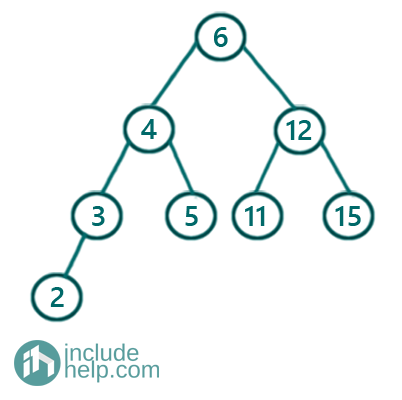5 is a dead end. Because you can't insert any value as a child of 5. For example, say we want to insert anything less than 2 that would be inserted as the left child of 2. 2, 3, 4 are already there. 6 is also there and anything more than 6 will be inserted in the right subtree. So basically no value can be inserted as a child of 5. So, it's a dead end. This end can't be extended anyhow.

So, it's clear that a BST can have a dead end. Now, the question is how we can check if there is a dead end or not.

So the idea is to check the range of elements we can insert. When the range becomes invalid then we can conclude that the BST has a dead end. No, of course initially the range for BST is [-INF, INF]. Now, say before insertion of any node the valid range is [l, r] then after the insertion of a node with value v under the constraint that v is in between the range [l, r], then the possible range for the left subtree of the inserted node will be [l, v-1] and the possible range for the right subtree will be [v+1, r]

For example,

Initially, the range is [-INF, INF] for the root

So, for its left subtree, the range would be [-INF, 5] and for its right subtree, it's [7, INF]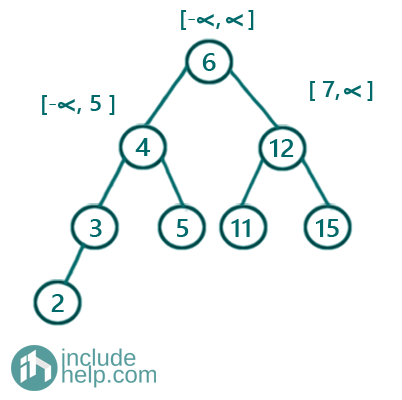Now, after checking the subtrees step by step we finally find the ranges associated with all the edges. Each edge contains a range which is the range for the connected child. After finding that, we can see that the edge b/w 4 to 5 has the range [5, 5] which means 5 is the only possible value of the child if there is any. Since 5 is the child, so if we think about the ranges for any possible left subtree for 5, that will be [5, 4] which is invalid and if we think about the ranges for any possible right subtree for 5, that will be [6, 5] which is again invalid. Thus 5 is a dead end.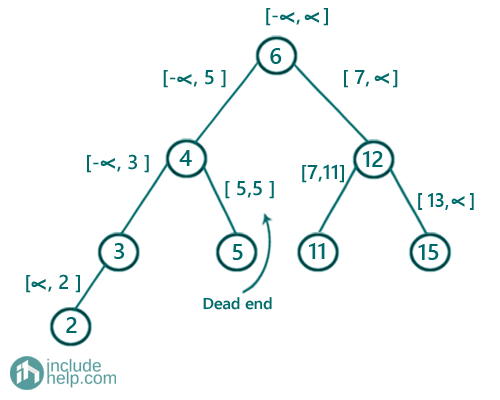Now to explore more, let me show you future possible dead ends. For example, there can't be any right subtree of 3. That you can think as a dead end too. Also, for Node 2, It can only have left subtrees and its right subtree is not possible, so that's another dead end. Similarly, for node 11, the right subtree is not possible and that's another future possible dead end. Future possible dead end means we can't have any subtree starting from there. Of course, these are not complete dead ends as the corresponding node is having another subtree possible or already existing.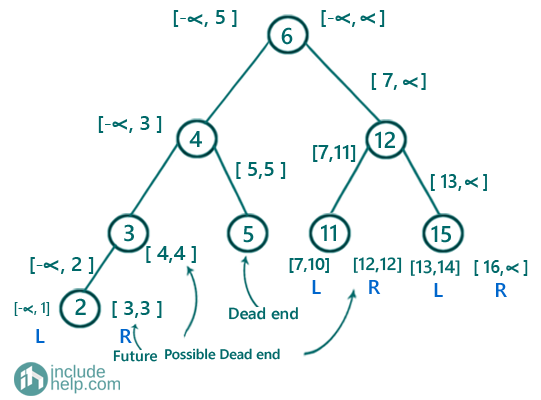Below is another example, where there is no dead end.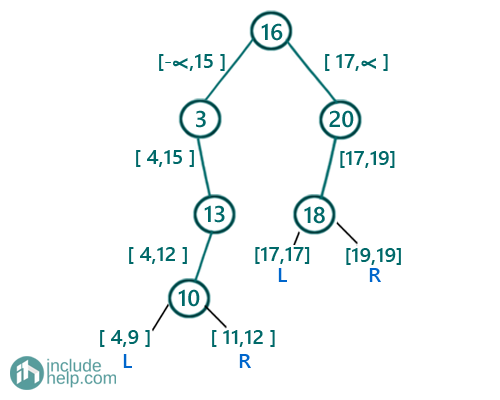So, here there is no dead end as 10 can have values in the range [4,9] as left subtree whereas [11,12] as a right subtree. Also, 18 can have values in the range [17, 17](17 is the only possible candidate) as a left subtree whereas [19,19](19 is the only possible candidate) as a right subtree. So there is no dead end in the above example, but of course, if we insert 18 or 19 then that will become a dead end as we saw in example 1.

```#include <bits/stdc++.h>
using namespace std;

// tree node is defined
class TreeNode {
public:
int val;
TreeNode* left;
TreeNode* right;
TreeNode(int data)
{
val = data;
left = NULL;
right = NULL;
}
};

//recursive function to check if there is a dead end
//return true if there is no dead end
//return false if there is dead end
bool isAnyDeadEndRecur(TreeNode* root, int range_min, int range_max)
{
//base case
//if root is NULL, no dead end
if (!root)
return true;

//invalid range so no further insertion possible
if (range_min >= range_max)
return false;

return isAnyDeadEndRecur(root->left, range_min, root->val - 1) && isAnyDeadEndRecur(root->right, root->val + 1, range_max);
}

//function to check if there is a dead end
//returns true if there is a dead end
//returns false if there is no dead end
{

// initially, the range is [INT_MIN, INT_MAX]
//retuned negation of the result from the recursive function
}

int main()
{
//tree same as the 1st example
TreeNode* root1 = new TreeNode(6);
root1->left = new TreeNode(4);
root1->right = new TreeNode(12);
root1->left->left = new TreeNode(3);
root1->left->left->left = new TreeNode(2);
root1->left->right = new TreeNode(5);
root1->right = new TreeNode(12);
root1->right->left = new TreeNode(11);
root1->right = new TreeNode(15);

if (result) {
cout << "There is dead end in the BST\n";
}
else {
cout << "There is no dead end in the BST\n";
}

//tree same as the 2nd example
TreeNode* root2 = new TreeNode(16);
root2->left = new TreeNode(3);
root2->left->right = new TreeNode(13);
root2->left->right->left = new TreeNode(10);
root2->right = new TreeNode(20);
root2->right->left = new TreeNode(18);

if (result) {
cout << "There is dead end in the BST\n";
}
else {
cout << "There is no dead end in the BST\n";
}

return 0;
}
```

Output:

```There is dead end in the BST
There is no dead end in the BST
```

Top MCQs

Languages: » C » C++ » C++ STL » Java » Data Structure » C#.Net » Android » Kotlin » SQL
Web Technologies: » PHP » Python » JavaScript » CSS » Ajax » Node.js » Web programming/HTML
Solved programs: » C » C++ » DS » Java » C#
Aptitude que. & ans.: » C » C++ » Java » DBMS
Interview que. & ans.: » C » Embedded C » Java » SEO » HR
CS Subjects: » CS Basics » O.S. » Networks » DBMS » Embedded Systems » Cloud Computing
» Machine learning » CS Organizations » Linux » DOS
More: » Articles » Puzzles » News/Updates